Electron. J. Diff. Equ., Vol. 2015 (2015), No. 279, pp. 1-6.

### Solutions to nonlinear Schrodinger equations for special initial data Takeshi Wada

Abstract:
This article concerns the solvability of the nonlinear Schrodinger equation with gauge invariant power nonlinear term in one space dimension. The well-posedness of this equation is known only for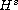with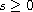. Under some assumptions on the nonlinearity, this paper shows that this equation is uniquely solvable for special but typical initial data, namely the linear combinations of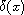and p.v. (1/x), which belongs to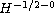. The proof in this article allows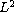-perturbations on the initial data.

Submitted March 27, 2015. Published November 10, 2015.
Math Subject Classifications: 35Q55.
Key Words: Nonlinear Schrodinger Equations; solvability; rough initial data.

Show me the PDF file (194 KB), TEX file, and other files for this article.Takeshi Wada Department of Mathematics Shimane University Matsue 690-8504, Japan email: wada@riko.shimane-u.ac.jp